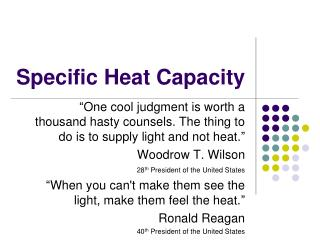DownloadDownload PresentationSpecific Heat Capacity

# Specific Heat Capacity

Télécharger la présentation## Specific Heat Capacity

- - - - - - - - - - - - - - - - - - - - - - - - - - - E N D - - - - - - - - - - - - - - - - - - - - - - - - - - -
##### Presentation Transcript

1. Specific Heat Capacity “One cool judgment is worth a thousand hasty counsels. The thing to do is to supply light and not heat.” Woodrow T. Wilson 28th President of the United States “When you can't make them see the light, make them feel the heat.” Ronald Reagan 40th President of the United States

2. Quantity of Heat • Heat energy (thermal energy) is measured in units called Joules (symbol: J). • Barbecues often quote their heat energy output in terms of British Thermal Units (btu). 1 J = 1055 Btu • This Napoleon Grill can produce 74,000 Btu (or about 70 J of heat energy)

3. Amount of Heat Energy • The amount of heat energy in a substance depends on several factors. Temperature (measured in degrees Celsius, oC) • Definition: The degree of hotness or coldness of a body or environment (corresponding to its molecular activity). • the higher the temperature of a fixed amount of a substance, the greater the heat energy present. • Example: A full bathtub with 42° water has more heat energy than a full bathtub with 20° water.

4. Amount of Heat Energy • The amount of heat energy in a substance depends on several factors. Mass (measured in kilograms, kg, or grams, g) • Definition: A measure of the quantity of matter. • the larger the mass, the greater the number of particles with heat energy. • Example: A full bathtub of 42° water has more heat energy than a 1/2 of a bathtub with 42° water. • NOTE: 1 kg = 1000 g

5. Amount of Heat Energy • The amount of heat energy in a substance depends on several factors. Substance Specific Properties • Different substances vary in their thermal properties. • Example: When you eat a hot pizza, you burn your tongue on the sauce not the crust. • Example: A metal spoon in a pot of boiling water will warm up faster than a wooden spoon in the same pot. • This thermal property is called specific heat capacity, and this also determines the amount of heat energy in a substance.

6. Specific Heat Capacity • Recall: • Heat is the energy that flows from one object to another due to a difference in their temperatures. • When heat flows into an object its thermal energy increases, as does its temperature. • Specific heat capacityis defined as the quantity of heat energy needed to raise the temperature of one gram of a substance by 1°C. Heat, like other energies, is measured in the unit, joules (J). In formula format specific heat capacity is:

7. Specific Heat Capacity • Substances with a low specific heat capacity warm quickly because they need less heat energy for a given change in temperature. They also give up their heat quickly. • Substances with a high specific heat capacity take a long time to warm up and they retain their heat for a long time.

8. Specific Heat Capacity • Symbolically we represent the amount of heat gained or lost as “Q”, the mass involved as “m”, the temperature change as “DT”, and the specific heat capacity as “c”. • Based on our definition of Specific Heat Capacity

9. Specific Heat Capacity • This relationship is usually written as where Q = heat energy (in Joules, J) m = mass (in grams, g) c = specific heat capacity (in J / g •oC) DT = Tf-Ti= temperature change (in oC)

10. The Specific Heat Capacity of Water • The specific heat capacity of water is 4.18 J / g • oC • This means that 4.18 J of heat energy are required to increase the temperature of a mass of 1 g of water by 1oC.